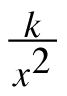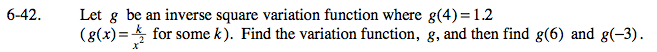### Home > PC > Chapter 6 > Lesson 6.1.3 > Problem6-42

6-42.

Let g be an inverse square variation function where g(4) = 1.2 (g(x) =for some k). Find the variation function, g, and then find g(6) and g(−3). Homework Help ✎Plug in the point (4,1.2) to find 'k' first.